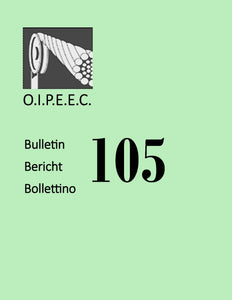# INFLUENCE OF LAY TYPES ON CURVATURE PERIOD OF DOUBLE SPIRAL WIRES IN WIRE ROPE USING DIFFERENTIAL GEOMETRY

• €0,00

Summary

The coordinate equation of the double spiral steel wires in wire rope is established by using differential geometry. In different lay types, the formula for calculating the ratio between rotation angle in wire and revolution angle in strand (denoted by n) of the double spiral steel wires with the same structure is given. Some expressions for n are derived mathematically.

Keywords: steel wire; lay type; double spiral steel wires; curvature; curvature cycle; differential geometry.

Author(s): Zhijun Shen, Wanxin Qin, Yuanbo You